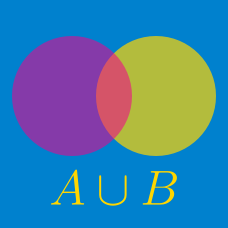Discrete Mathematics

# Sets - Relative Complement

If $$A$$ and $$B$$ are subsets of the set $$U=\{0,3,5,10,12,16,18\}$$ such that $A \cap B^{C}=\{3,5\}, A^{C} \cap B=\{10,12\}, A^{C} \cap B^{C}=\{16,18\},$ then which of the following is equal to $$A \cap B?$$

Details and assumptions

You may choose to read the summary page Set Notation.

Consider the ground set $U=\{x \mid x \mbox{ is a positive integer } \leq 17\}.$ If $$A=\{1,2,\ldots,5\}$$ and $$B$$ is a subset of $$U$$ such that $A \cap B=\{4, 5\}, (U \setminus A) \cap (U \setminus B)=\{16, 17\},$ what is $$\lvert B \rvert?$$

Consider the ground set $U=\{x \mid x \mbox{ is a positive integer } \leq 35\}$ and let $$A$$ and $$B$$ be two subsets of $$U$$ such that $A=\{1, 2, 3, \ldots, 17\}, \; A \setminus(A \setminus B)=A.$ What is $$\lvert (U \backslash A) \cup B \rvert?$$

Details and assumptions

You may choose to read the summary page Set Notation.

Consider the sets $A=\{x \mid 3 \leq x < 50\}, B=\{x \mid x \leq 0 \text{ or } x > 43\}.$ How many integers are there in the set $$A \setminus B?$$

Details and assumptions

You may choose to read the summary page Set Notation.

Consider a ground set $$U$$ and let $$A$$ and $$B$$ be two subsets of $$U.$$ If $\lvert U \rvert=70, \lvert A \rvert=37, \lvert A \cap B \rvert=13, \lvert A \cup B \rvert=48,$ what is $$\lvert U \setminus B^c \rvert?$$

Details and assumptions

You may choose to read the summary page Set Notation.

×

Problem Loading...

Note Loading...

Set Loading...